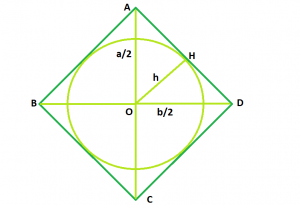# Area of circle inscribed within rhombus

Given a rhombus with diagonals a and b, which contains an inscribed circle. The task is to find the area of that circle in terms of a and b.

Examples:

```Input: l = 5, b = 6
Output: 11.582

Input: l = 8, b = 10
Output: 30.6341
```## Recommended: Please try your approach on {IDE} first, before moving on to the solution.

Approach: From the figure, we see, the radius of inscribed circle is also a height h=OH of the right triangle AOB. To find it, we use equations for triangle’s area :

Area AOB = 1/2 * (a/2) * (b/2) = ab/8 = 12ch

where c = AB i.e. a hypotenuse. So,

r = h = ab/4c = ab/4√(a^2/4 + b^2/4) = ab/2√(a^2+b^2)

and therefore area of the circle is

A = Π * r^2 = Π a^2 b^2 /4(a2 + b2)

Below is the implementation of above approach:

## C++

 `// C++ Program to find the area of the circle ` `// which can be inscribed within the rhombus ` `#include ` `using` `namespace` `std; ` ` `  `// Function to find the area ` `// of the inscribed circle ` `float` `circlearea(``float` `a, ``float` `b) ` `{ ` ` `  `    ``// the diagonals cannot be negative ` `    ``if` `(a < 0 || b < 0) ` `        ``return` `-1; ` ` `  `    ``// area of the circle ` `    ``float` `A = (3.14 * ``pow``(a, 2) * ``pow``(b, 2)) ` `            ``/ (4 * (``pow``(a, 2) + ``pow``(b, 2))); ` `    ``return` `A; ` `} ` ` `  `// Driver code ` `int` `main() ` `{ ` `    ``float` `a = 8, b = 10; ` `    ``cout << circlearea(a, b) << endl; ` ` `  `    ``return` `0; ` `} `

## Java

 `// Java Program to find the area of the circle  ` `// which can be inscribed within the rhombus  ` ` `  `public` `class` `GFG { ` `     `  `    ``// Function to find the area  ` `    ``// of the inscribed circle  ` `    ``public` `static` `float` `circlearea(``double` `a, ``double` `b) ` `    ``{ ` `        ``// the diagonals cannot be negative ` `        ``if` `(a < ``0` `|| b < ``0``) ` `            ``return` `-``1` `; ` `         `  `        ``//area of the circle  ` `        ``float` `A = (``float``) ((``3.14` `* Math.pow(a, ``2``) * Math.pow(b, ``2``))  ` `                        ``/ (``4` `* (Math.pow(a, ``2``) + Math.pow(b, ``2``)))) ; ` `         `  `        ``return` `A ; ` `    ``} ` ` `  `    ``// Driver code ` `    ``public` `static` `void` `main(String[] args) { ` `        ``float` `a = ``8``, b = ``10` `; ` `         `  `        ``System.out.println(circlearea(a, b)); ` ` `  `    ``} ` `// This code is contributed by ANKITRAI1 ` `} `

## Python 3

 `# Python 3 Program to find the area of the circle ` `# which can be inscribed within the rhombus ` ` `  ` `  `# Function to find the area ` `# of the inscribed circle ` `def` `circlearea(a, b): ` ` `  `    ``# the diagonals cannot be negative ` `    ``if` `(a < ``0` `or` `b < ``0``): ` `        ``return` `-``1` ` `  `    ``# area of the circle ` `    ``A ``=` `((``3.14` `*` `pow``(a, ``2``) ``*` `pow``(b, ``2``))``/` `        ``(``4` `*` `(``pow``(a, ``2``) ``+` `pow``(b, ``2``)))) ` `    ``return` `A ` ` `  `# Driver code ` `if` `__name__ ``=``=` `"__main__"``: ` `    ``a ``=` `8` `    ``b ``=` `10` `    ``print``( circlearea(a, b)) ` ` `  `# This code is contributed by ChitraNayal `

## C#

 `// C# Program to find the area of the circle  ` `// which can be inscribed within the rhombus ` `using` `System; ` ` `  `public` `class` `GFG { ` `     `  `    ``// Function to find the area  ` `    ``// of the inscribed circle  ` `    ``public` `static` `float` `circlearea(``double` `a, ``double` `b) ` `    ``{ ` `        ``// the diagonals cannot be negative ` `        ``if` `(a < 0 || b < 0) ` `            ``return` `-1 ; ` `         `  `        ``//area of the circle  ` `        ``float` `A = (``float``) ((3.14 * Math.Pow(a, 2) * Math.Pow(b, 2))  ` `                        ``/ (4 * (Math.Pow(a, 2) + Math.Pow(b, 2)))) ; ` `         `  `        ``return` `A ; ` `    ``} ` ` `  `    ``// Driver code ` `    ``public` `static` `void` `Main() { ` `        ``float` `a = 8, b = 10 ; ` `         `  `        ``Console.WriteLine(circlearea(a, b)); ` ` `  `    ``} ` `// This code is contributed by inder_verma.. ` `} `

## PHP

 ` `

Output:

```30.6341
```

Attention reader! Don’t stop learning now. Get hold of all the important DSA concepts with the DSA Self Paced Course at a student-friendly price and become industry ready.

My Personal Notes arrow_drop_upCheck out this Author's contributed articles.

If you like GeeksforGeeks and would like to contribute, you can also write an article using contribute.geeksforgeeks.org or mail your article to contribute@geeksforgeeks.org. See your article appearing on the GeeksforGeeks main page and help other Geeks.

Please Improve this article if you find anything incorrect by clicking on the "Improve Article" button below.

Article Tags :
Practice Tags :

Be the First to upvote.

Please write to us at contribute@geeksforgeeks.org to report any issue with the above content.